# It seems to be a problem in the instructions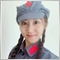97

```void OnStart()
{
uchar  u_ch;

for(char ch=-128;ch<=127;ch++)
{
u_ch=ch;
Print("ch = ",ch," u_ch = ",u_ch);
if(ch==127) break;
}
}```

When executing the program, the first time u_ch=ch,      ch=-128,u_ch=0.

However, when printing, ch= -128 u_ch= 128.

Why is u_ch not 127?It should be 255-128=127 when printing, right?

```ch= -128  u_ch= 128
ch= -127  u_ch= 129
ch= -126  u_ch= 130
ch= -125  u_ch= 131
ch= -124  u_ch= 132
ch= -123  u_ch= 133
ch= -122  u_ch= 134
ch= -121  u_ch= 135
ch= -120  u_ch= 136
ch= -119  u_ch= 137
ch= -118  u_ch= 138
ch= -117  u_ch= 139
ch= -116  u_ch= 140
ch= -115  u_ch= 141
ch= -114  u_ch= 142
ch= -113  u_ch= 143
ch= -112  u_ch= 144
ch= -111  u_ch= 145
... ```19633

helloea: Why is u_ch not 127?It should be 255-128=127 when printing, right?

No, because it has nothing to do with subtraction.

 255 (decimal) as unsigned is FF (hex) or 1111 1111 (binary)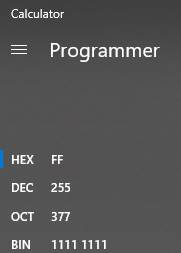As signed, the high bit means negative.The remaining bits mean 7F (hex) or 0111 1111 (binary) or 127 decimal.Thus it is minus 127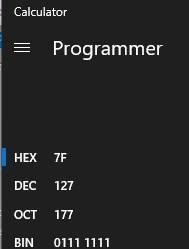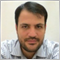2484

helloea:

However, when printing, ch= -128 u_ch= 128.

Why is u_ch not 127?It should be 255-128=127 when printing, right?

It is related to binary representation of char and uchar.

-128(char) is 1000 0000(BIN), the equivalent is 128(uchar) with the same bits: 1000 0000(BIN).

In this situation a typecasting is not performed, but the memory block is copied.

Typecasting is performed like the following scheme: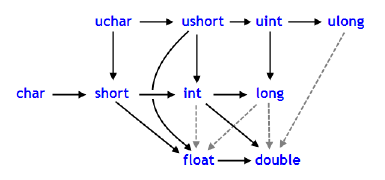According to documentation:

Conversions between char and uchar, short and ushort, int and uint, long and ulong (conversions to both sides), may lead to the loss of data.